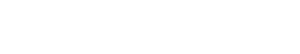# Time Series

The term “Time Series” generally refers to a sequence of data points, usually measurements or observations, that are collected at regular intervals. Time series data is often used for predictive modeling and forecasting, since it can provide insights about future trends based on past patterns.

Outside of the text analytics industry, the term “Time Series” may have a different definition. For example, in finance, a time series may be a sequence of stock prices over time. In statistics, a time series may be a sequence of observations of some phenomenon (e.g., temperature changes) over time.

So, when we talk about Time Series in the context of text analytics, we are referring to a sequence of data points that are related to each other in some way and are collected over time. This data can be used to predict future trends based on past patterns.

Time Series is sometimes confused with other similar terms, such as “time-series analysis” and “time-series data.” Time-series analysis is a statistical technique that can be used to analyze time series data. Time-series data is simply data that is collected over time. So, while time series and time-series data are related, they are not the same thing.

## Uses of Time Series Data

Time series data is often used for predictive modeling and forecasting. This is because time series data can provide insights about future trends based on past patterns. For example, if we have a time series of monthly sales data, we can use that data to predict future sales patterns. Time series data can also be used to detect anomalies (e.g., spikes or dips in sales) and to understand how different factors (e.g., seasonality, events, etc.) impact the phenomenon being observed.

There are many different ways to analyze time-series data. Some common methods include:

• Visualization: Time series data can be visualized using line graphs, bar charts, or other types of graphs. This can help you to identify patterns or trends in the data.
• Statistical techniques: There are many statistical techniques that can be used to analyze time-series data. Some common techniques include regression analysis, time-series analysis, and autoregressive moving average (ARMA) models.
• Machine learning: Machine learning algorithms can be used to automatically identify patterns in time series data. This is often used for predictive modeling and forecasting.Unlock the power of actionable insights with AI-based natural language processing.